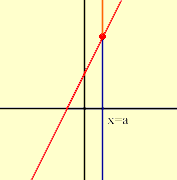Who is asking: Student Level: Secondary Question: how can I solve and graph y < 2x+1 and which side in the graph should i shade after i finish solving my inequality? Hi Erika, I drew a sketch of the line y = 2x + 1.Now consider some x-cordinate, say x = a and look at all the points in the plane with x-cordinate a. Some of these points are below the line, some are above the line and one is on the line.In my diagram the points in blue have x=a and are below the line, the points in orange have x=a and are above the line, and the red dot has x=a and is on the line. Since this red dot is on the line and its x-coordinate is a, its y-coordinate must be 2a+1. The blue points must then have y-coordinates less than 2a+1, that is the blue points satisfy y < 2x+1. Hence the part of the plane that satisfies y < 2x+1 is the region below the line y = 2x+1. Penny Go to Math Central